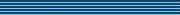| home      | people     | research     | publications    | seminars    | events     | contactCommunication Networks   | Systems Biology   | Hybrid Systems    | Machine Learning   | Dynamics & Interaction### Schools Mathematics Grand Challenge

Week eight's Puzzles

Problem 22:

The solution was:

The answer is 5000000050000000. To get the answer we use a trick. The trick is to observe that

2 / ((k+1)*(k+2)*(k+3)) = 1/((k+1)*(k+2)) - 1/((k+2)*(k+3))

for all k ≥ 0. This allows us to write:

2A = [1/(1*2) - 1/(2*3)] + [1/(2*3) - 1/(3*4)] + [1/(3*4) - 1/(4*5)] + ... etc.

After rearranging the brackets we get:

2A = 1/(1*2) + [1/(2*3)-1/(2*3)] + [1/(3*4)-1/(3*4)] + ... - = 1/(1000000*1000001)

since the terms between the brackets are all zero it follows that

2A = 1/(1*2) - 1/(1000000*1000001),

which gives us:

1/(1-4A) = 5000000050000000.

We also accepted answers close to 6 because there was a typo in the original question, which would have given an answer of almost 6.

Problem 23:

The solution was:

It seems obvious to use 1,2,4,8 as one of the geometric sequences, as it is the only geometric sequence covering 4 of our numbers. What other sequences will cover lots of balls? Note that we can make a geometric sequence hit any two numbers a and b by choosing the sequence to be a, b = a*(b/a), a*(b/a)*(b/a), etc. If we do this with numbers where a does not divide into b however, only two of the terms will be whole numbers.

So a solution is 1,2,4,8 then 3,5 then 6,7 and then 9; so Jo can do it with four sequences. If the first sequence is 1,2,4,8 then the remaining numbers are 3,5,6,7,9. By looking at all the pairs of these numbers, we can check that a sequence with any pair will not also contain any of the remaining numbers. That means that if we start with 1,2,4,8 then the best we can do is pick off pairs, which means we'll always need 4.

The final thing to do is check that not using 1,2,4,8 doesn't help enough to get the total down to three. If we have three sequences covering 9 numbers, and none of them hit 4 (or more) numbers, then all three sequences must hit three numbers. By checking the possibilities, we can see that is impossible. So, 4 sequences is the best we can do.

Problem 24:

The solution was:

This very hard problem is called the problem of Derangements. Induction is one of several ways to solve this problem. Here we will use the method of Inclusion-Exclusion, where we first double count some things and then go back and correct ourselves.

Let's begin with ABCD to get the idea. There are 4*3*2*1 = 24 ways to arrange these letters:

 ABCD BACD CABD DABC ABDC BADC CADB DACB ACBD BCAD CBAD DBAC ACDB BCDA CBDA DBCA ADBC BDAC CDAB DCAB ADCB BDCA CDBA DCBA

How many have at least one letter in the correct place? There are 15. The number of left over ones (9) is the number we want, the number that are all completely misspelled. How can we work out 15 without just counting them?

We can ESTIMATE the number that has at least one letter in the correct place as the sum of the number that have A in the correct place (6) plus the number that have B in the correct place (6) etc. This will gives us 24, which is WRONG, but is a start. What did we do wrong?

We counted words like ABDC too many times, once for the A in the correct place, once for the B in the correct place etc. We have to correct 24 by subtracting off the number of words that have at least A and B in the correct place (2), then the number that have at least A and C in the correct place (2), then the number that have at least A and D in the correct place (2), then the number that have at least B and C in the correct place (2), etc. This all adds up to 12. Our estimate is now 24-12 = 12, which is still wrong, but a lot closer to 15!!!

Actually we have subtracted off too much, and have to add back in the number that have at least A and B and C in the correct place (1), and the number that have at least A and B and D in the correct place (1), and the number that have at least A and C and D in the correct place (1), etc. This all adds up to 4, and we are up to 24-12+4 = 16. Now finally we added back in the number that have A and B and C and D in the correct place too many times (1) and have to subtract that off to get 15. Phew!!!

You can check that the totals in each case always follows the pattern 4*3*2*1 or 4*3*2 or 4*3 or 4 or 1. So, we get 15 = 4*3*2*1 - 4*3 + 4 - 1 and the answer is 9 = 24 - 4*3*2*1+4*3-4+1.

The same pattern works for ALGORITHM starting with 10; the answer is 1334961 = 10*9*8*7*6*5*4*3*2*1 - 10*9*8*7*6*5*4*3*2*1 + 10*9*8*7*6*5*4*3 - 10*9*8*7*6*5*4 + 10*9*8*7*6*5 - 10*9*8*7*6 + 10*9*8*7 - 10*9*8 + 10*9 - 10 + 1.